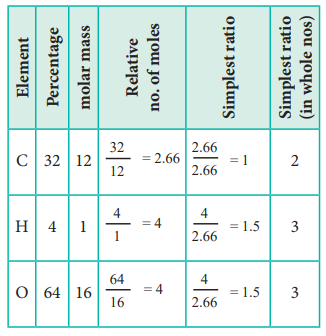Home | | Chemistry 11th std | Determination of Empirical Formula from Elemental Analysis Data

# Determination of Empirical Formula from Elemental Analysis Data

Determination of Empirical Formula from Elemental Analysis Data

Determination of Empirical Formula from Elemental Analysis Data :

Step 1:  Since   the   composition   is expressed  in  percentage,  we can consider the total mass of the compound as 100 g and the percentage values of individual elements as mass in grams.

Step 2:  Divide the mass of each element by its atomic mass. This gives the relative number of moles of various elements in the compound.

Step 3:  Divide the value of relative number of moles obtained in the step 2 by the smallest number of them to get the simplest ratio.

Step 4:  (only if necessary) in case the simplest ratios obtained in the step 3 are not whole numbers then they may be converted into whole number by multiplying by a suitable smallest number.

Example:

1. An acid found in tamarinds on analysis shows the following percentage composition: 32 % Carbon; 4 % Hydrogen; 64 % Oxygen. Find the empirical formula of the compound.The empirical formula is C2H3O3

2. An organic compound present in vinegar has 40 % carbon, 6.6 % hydrogen and 53.4 % oxygen. Find the empirical formula of the compound.The empirical formula is CH2O

Molecular formula of a compound is a whole number multiple of the empirical formula. The whole number can be calculated from the molar mass of the compound using the following expressionStudy Material, Lecturing Notes, Assignment, Reference, Wiki description explanation, brief detail
11th Chemistry : UNIT 1 : Basic Concepts of Chemistry and Chemical Calculations : Determination of Empirical Formula from Elemental Analysis Data |